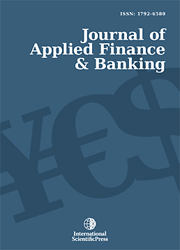# Journal of Applied Finance & Banking

#### Portfolio Selection Using Genetic Algorithm

•[ Download ]
• Times downloaded: 3504
• Abstract

The selection of optimal portfolios is the central problem of financial investment decisions. Mathematically speaking, portfolio selection refers to the formulation of an objective function that determines the weights of the portfolio invested in each asset as to maximize return and minimize risk. This paper applies the method of genetic algorithm (GA) to obtain an optimal portfolio selection. However, the GA parameters are of great importance in the procedure of convergence of this algorithm towards the optimal solution such as crossover. While, a five stock portfolio example is used in this paper to illustrate the applicability and efficiency of genetic algorithm method, GA method can also be used however for a larger number of portfolio compositions. The results obtained confirm previous research studies about the validity and efficiency of genetic algorithm in selecting optimal portfolios.ISSN: 1792-6599 (Online)
1792-6580 (Print)